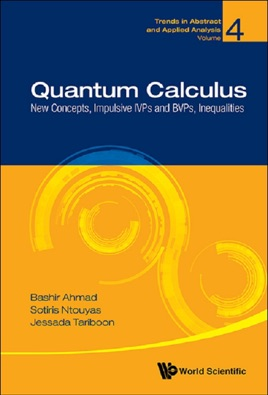• USD 37.99

## Descripción de editorial

The main objective of this book is to extend the scope of the q-calculus based on the definition of q-derivative [Jackson (1910)] to make it applicable to dense domains. As a matter of fact, Jackson's definition of q-derivative fails to work for impulse points while this situation does not arise for impulsive equations on q-time scales as the domains consist of isolated points covering the case of consecutive points. In precise terms, we study quantum calculus on finite intervals.

In the first part, we discuss the concepts of qk-derivative and qk-integral, and establish their basic properties. As applications, we study initial and boundary value problems of impulsive qk-difference equations and inclusions equipped with different kinds of boundary conditions. We also transform some classical integral inequalities and develop some new integral inequalities for convex functions in the context of qk-calculus. In the second part, we develop fractional quantum calculus in relation to a new qk-shifting operator and establish some existence and qk uniqueness results for initial and boundary value problems of impulsive fractional qk-difference equations.
Contents:PreliminariesQuantum Calculus on Finite IntervalsInitial Value Problems for Impulsive qk-Difference Equations and InclusionsBoundary Value Problems for First-Order Impulsive qk-Integro-Difference Equations and InclusionsImpulsive qk-Difference Equations with Different Kinds of Boundary ConditionsNonlinear Second-Order Impulsive qk-Difference Langevin Equation with Boundary ConditionsQuantum Integral Inequalities on Finite IntervalsImpulsive Quantum Difference Systems with Boundary ConditionsNew Concepts of Fractional Quantum Calculus and Applications to Impulsive Fractional qk-Difference EquationsIntegral Inequalities via Fractional Quantum CalculusNonlocal Boundary Value Problems for Impulsive Fractional qk-Difference EquationsExistence Results for Impulsive Fractional qk-Difference Equations with Anti-periodic Boundary ConditionsImpulsive Fractional qk-Integro-Difference Equations with Boundary ConditionsImpulsive Hybrid Fractional Quantum Difference Equations